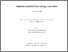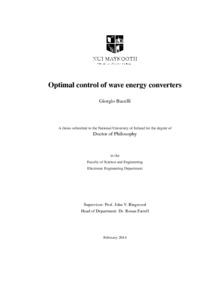# Optimal control of wave energy converters

Bacelli, Giorgio (2014) Optimal control of wave energy converters. PhD thesis, National University of Ireland Maynooth.Previewmore...Add this article to your Mendeley library

## Abstract

Wave Energy Converters (WECs) are devices designed to absorb energy from ocean waves. The particular type of Wave Energy Converter (WEC) considered in this thesis is an oscillating body; energy conversion is carried out by means of a structure immersed in water which oscillates under forces exerted by waves. This thesis addresses the control of oscillating body WECs and the objective of the control system is to optimise the motion of the devices that maximises the energy absorption. In particular, this thesis presents the formulation of the optimal control problem for WECs in the framework of direct transcription methods, known as spectral and pseudospectral optimal control. Direct transcription methods transform continuous time optimal control problems into Non Linear Programming (NLP) problems, for which the literature (and the market) offer a large number of standard algorithms (and software packages). It is shown, in this thesis, that direct transcription gives the possibility of formulating complex control problems where realistic scenarios can be taken into account, such as physical limitations and nonlinearities in the behaviour of the devices. Additionally, by means of spectral and pseudospectral methods, it is possible to find an approximation of the optimal solution directly from sampled frequency and impulse response models of the radiation forces, obviating the need for finite order approximate models. By implementing a spectral method, convexity of the NLP problem, associated with the optimal control problem for a single body WEC described by a linear model, is demonstrated analytically. The solution to a nonlinear optimal control problem is approximated by means of pseudospectral optimal control. In the nonlinear case, simulation results show a significant difference in the optimal behaviour of the device, both in the motion and in the energy absorption, when the quadratic term describing the viscous forces are dominant, compared to the linear case. This thesis also considers the comparison of two control strategies for arrays of WECs. A Global Control strategy computes the optimal motion by taking into account the complete model of the array and it provides the global optimum for the absorbed energy. In contrast, an Independent Control strategy implements a control system on each device which is independent from all the other devices. The final part of the thesis illustrates an approach for the study of the effects of constraints on the total absorbed energy. The procedure allows the feasibility of the constrained energy maximisation problem to be studied, and it provides an intuitive framework for the design of WECs relating to the power take-off operating envelope, thanks to the geometrical interpretation of the functions describing both the total absorbed energy and the constraints.

Item Type: Thesis (PhD) Optimal control; wave energy converters; Faculty of Science and Engineering > Electronic Engineering 6753 IR eTheses 08 Jan 2016 14:40Item control page